# Summerjob

Three students participated in the summerjob. Altogether they earn 1780, -. Peter got a third less than John and Paul got 100,- more than Peter. How much got every one of them?

Correct result:

a =  480
b =  720
c =  580

#### Solution:

a+b+c=1780
a=b-b/3
c=100+a

a+b+c = 1780
3a-2b = 0
a-c = -100

a = 480
b = 720
c = 580

Our linear equations calculator calculates it.We would be pleased if you find an error in the word problem, spelling mistakes, or inaccuracies and send it to us. Thank you!

Showing 1 comment:Smarty
Too hard to find out the answer!Tips to related online calculators
Do you have a linear equation or system of equations and looking for its solution? Or do you have quadratic equation?

## Next similar math problems:

• Peter and PaulPeter and Paul together have 26 years. Four years ago, Paul was twice older than Peter. How much is Paul and how much Peter?
• Paul earnedPaul earned 300 Kč in one hour, Václav 1/3 more than Paul. Václav worked 60 hours, which is 1/3 fewer hours than Paul worked. How many percents less earned Paul an hour than Václav? How many hours did Paul more than Václav? How much did Paul earn more tha
• Boys and money270 USD boys divided so that Peter got three times more than Paul and Ivan has 120 USD more than than Paul. How much each received?
• SchoolsThree schools are attended by 678 pupils. To the first attend 21 students more and to the third 108 fewer students than to second school. How many students attend the schools?
• Summerjob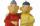Albert, Beny, Cyril and Dan worked on summerjob. From the total revenue Albert received 2/5, Beny 1/6 , 3/10 Cyril and Dan the rest which is 324 euros. How many euros earned every one of them?
• Jane classWhen asked how many students are in class, Jane said, if we increase the number of students in our class by hundred % and then add half the number of students, we get 100. How many students are in Jane's class?
• Three friendsThe three friends spent 600 KC in a teahouse. Thomas paid twice as much as Paul. Paul a half less than Zdeněk. How many each paid?
• 13 ticketsA short and long sightseeing tour is possible at the castle. Ticket for a short sightseeing circuit costs CZK 60, for a long touring circuit costs CZK 100. So far, 13 tickets have been sold for 1140 CZK. How much did you pay for tickets for a short tour?
• Saving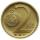Paul save 155Kč in 46 2 Kc and 5Kc coins. How much saved 2Kc and 5Kc coins?
• BanknotesEva deposit 7800 USD in 50 banknotes in the bank. They had value 100 USD and 200 USD. How many were they?
• Soccer balls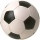Pupils in one class want to buy two soccer balls together. If each of them brings 12.50 euros, they will miss 100 euros, if each brings 16 euros, they will remain 12 euros. How many students are in the class?
• EurosPeter, Jane and Thomas have together € 550. Tomas has 20 euros more than Jane, Peter € 150 less than Thomas. Determine how much has each of them.
• Paul and Honza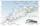Honza went to chat on a bike without a break 4.0 hours. Paul the same way without breaks 50 minutes longer. The difference between the speeds of the two boys was the one kilometer per hour. How fast was Paul, if you know that the speed of the boys did not
• Summer camp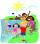Out of the 180 students at a summer camp, 72 signed up for canoeing. Twenty-three students signed up for trekking, and 13 of those students also signed up for canoeing. Use a two-way table to organize the information and answer the following question: App
• Two numbersWe have two numbers. Their sum is 140. One-fifth of the first number is equal to half the second number. Determine those unknown numbers.
• MoneyPeter has 2x more money than Michael. But if Peter gave Michael € 16 Michael would have 3.5 times more money than Peter. Determine how much money was originally Peter and how much Michael.
• Holiday SMS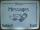Kucera and Malek start from the holiday together. They agreed that if they be 100 km apart, will send a SMS. Kucera traveling at 60 km/h., Malek 90 km/h. They started at 14 hours PM. At what time they send a message?